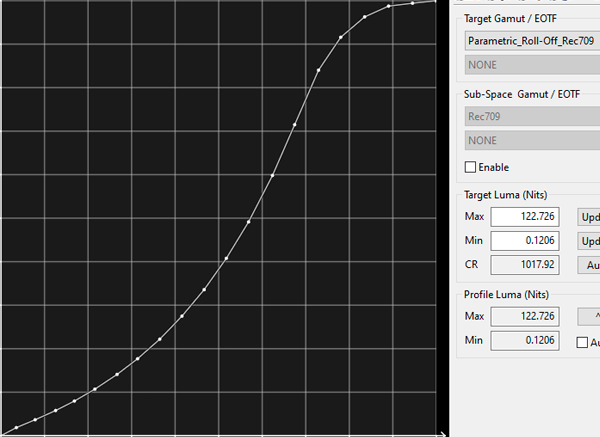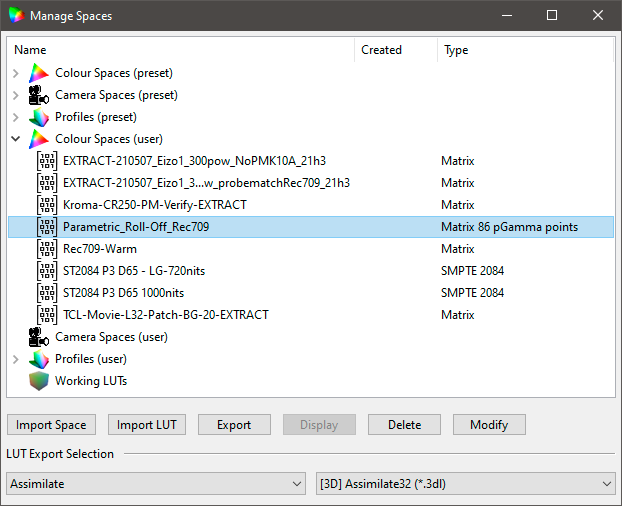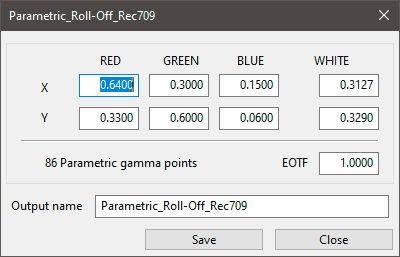Any colour space can have Parametric EOTF Controls added to it, enabling non-power law variable EOTF profiles to be defined by the user.

A Parametric EOTF can be defined with multiple adjustment points, and can help with displays and projectors that clip image detail in the blacks when a standard power law gamma is used, or to roll-off highlight detail, etc. With Parametric EOTF the user has full control over the target colour space EOTF/Gamma.

### Adding Parametric EOTF

Any colour space can have Parametric EOTF added to it, enabling non-power law variable gamma profiles to be defined.

To add a Parametric EOTF first save the basic colour space to be modified with a custom name, and then export via the Space library. Open the colour space .bcs file in any text editor and add the required Parametric Gamma variables using the following standard.

1. <?xml version="1.0" encoding="UTF-8" ?>
2. <builder_color_space name="Rec709_2.4_Para_Lift_Near_Black" version="2">
3. <head>
4. <x red="0.64" green="0.3" blue="0.15" white="0.3127" />
5. <y red="0.33" green="0.6" blue="0.06" white="0.329" />
6. <L red="1" green="1" blue="1" white="1" />
7. <gamma>2.400000</gamma>
8. <para points="4">
9. <point idx="0">
10. <ig>0.000000</ig>
11. <og>0.000000</og>
12. </point>
13. <point idx="1">
14. <ig>0.030000</ig>
15. <og>0.036000</og>
16. </point>
17. <point idx="2">
18. <ig>0.060000</ig>
19. <og>0.060000</og>
20. </point>
21. <point idx="3">
22. <ig>1.000000</ig>
23. <og>1.000000</og>
24. </point>
25. </para>
26. </head>
27. </builder_color_space>

The section <para> is used to define the Parametric EOTF points. If used it must contain a minimum of 3 points, and the first and last points MUST be 0.0 and 1.0. The in-between values are normalized between the first and last values of 0.0 and 1.0.

The <point> values are differential change values, and will modify the underlying EOFT value. Therefore, the result for the same Parametric <point> values will vary with different underlying <gamma> value.

There can be as many points as needed in the list, and they must be monotonically increasing (for both ig and og). The recommendation is to not exceed 101 pairs.

The <gamma>2.400000</gamma> option defines the underlying colour space EOTF, and any <para points="xx"> values define an offset to the underlying EOTF value. Therefore a <gamma>1.0000</gamma> value would only use the provided Parametric EOTF values.
Note: as Parametric EOTF values are simply a change to the underlying <gamma> value, if <gamma> is set to 1.0 each Parametric <point> value will be connected linearly.

The above example has a very slight Near Black Lift at 3% of range, returning to the underlying EOTF at 6%, aimed at overcoming the near black clipping experienced with many LG WOLEDs.

In the above graphs the target EOTF is the grey line, and shows that the point at 0.03 is above the pure Rec709 2.4 Gamma plot in blue.
(The EOTF graph is zoomed-in.)

The difference in the Parametric EOTF can also be seen in the Target Y value vs. the pure Rec709 value, where a differece of 0.01 nits can be seen.

In the Spaces Library, text is displayed defining the Parametric Points for any colour space with a defined Parametric EOTF.Parametric EOTF LibraryParametric EOTF Modify

As above, a example of Parametric EOTF use can be to slightly lift near black on TVs that suffer black clipping, such as LG WOLEDs. For such an application a Parametric EOFT based on the target EOFT (2.4 for Rec709, for example) would first be generated, and then the near black points adjusted to slightly lift the target EOTF.

A couple of example colour spaces, with parametric gamma curves, can be downloaded via the Customer Downloads or General Downloads page.

The colour spaces can be loaded into ColourSpace for direct use, or opened via Notepad, or similar, so the data entries can be reviewed and edited.